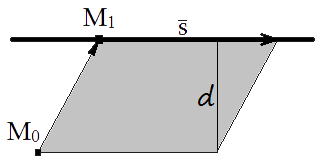Study of mathematics online.
Study math with us and make sure that "Mathematics is easy!"

# Online calculator. Distance from a point to a line - 3-Dimensional

This online calculator will help you to find distance from a point to a line in 3D.

Using this online calculator, you will receive a detailed step-by-step solution to your problem, which will help you understand the algorithm how to find distance from a point to a line in 3D.

## Distance from a point to a line 3D calculatorSelect the form of line representation:

Line equation:

x - = y - = z -

Point coordinates:

M0: (
,,
)

You can input only integer numbers, decimals or fractions in this online calculator (-2.4, 5/7, ...). More in-depth information read at these rules.